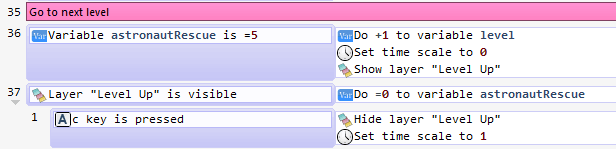# Change a level using variables

In my game, the player gets to the next level when the variable “astronautRescue” = 5. This is working, but I always have to reset the “astronautRescue” = 0. I do not want to reset this variable, but if I don’t do it, my next level does not work. Can you please suggest how to code this better? Thank you.Do you want to keep “astronautRescue”, so in the first level you should reach 5, in the second level reach 10, in the third 15… ?You could make the “astronautRescue” a function of “level”, or any another variable that changes between levels.
For example, in the level 1, you need “astronautRescue” = 5, and in the level 2, “astronautRescue” = 10, so you just do:

``If variable "astronautRescue" = "Variable(level) * 5"   |   Increase level, show layer, set time scale,etc.``

The nice thing in “functionalize” values is that it gives you not only linear equations, but any kind of equation, for example RPG games use such kind of functions (really a serie of them, algorithms) to calculate states and experience in function of the level. This funtion: 100*​e^​(x/​10)-​99 gives you a curve that smoothly increase over time, of course 100 is a constant to make the exponential doesn’t grow too fast, the 10 to get a more “linear” curve, and 99 to have at x=0 ==> y=1If this isn’t what you are asking for, excuse me, explain it and I’ll help you (anyway I think it’s interesting)I plugged in your code, and it worked exactly how I wanted! So easy! Thank you very much, Lizard-13!